## The SABR model: explicit formulae of the moments of the forward prices/rates variable and series expansions of the transition probability density and of the option prices

1. Abstract
2. The SABR model and the moment formulae
3. The moments of the forward prices/rates variable: an interactive application and an app (interactive application, app).
4. Calibration and option price forecast

.

1.    Abstract

In  the SABR stochastic volatility model with b-volatility b Î (0,1)  and an absorbing barrier in zero imposed to the forward prices/rates stochastic process is studied. The model is considered in presence of (possibly) nonzero correlation between the stochastic differentials that appear on the right hand side of the dynamic equations. A series expansion of the probability density function of the model in powers of the correlation coefficient of these stochastic differentials is presented. Explicit formulae for the first three terms of this expansion are derived. These formulae are integrals of known integrands. The zero-th order term of the expansion is a new integral formula containing only elementary functions of the transition probability density function of the SABR model when the correlation coefficient is zero. The expansion is deduced from the final value problem for the backward Kolmogorov  equation  satisfied by the transition probability density function. Each term of the expansion is defined as the solution of a final value problem for a partial differential equation. The integral formulae that give the solutions of these final value problems are based on the Hankel and on the Kontorovich-Lebedev transforms. From the series expansion of the probability density function we deduce the corresponding expansions of the  European call and put option prices. Moreover we deduce closed form formulae for the moments of the forward prices/rates variable. The moment formulae do not contain integrals or series expansions and are expressed using only elementary functions. The option pricing formulae are used to study synthetic and real data. In particular we study a time series of futures prices of the EUR/USD currency's exchange rate and of some of the corresponding  option prices. A general reference to the work of the authors and of their coauthors in mathematical finance is  the website: http://www.econ.univpm.it/recchioni/finance.

## 2. The SABR model and the moment formulae

The SABR model is a stochastic volatility model widely used in mathematical finance and in the practice of the financial markets to describe the dynamics of the forward prices/rates variable and of its stochastic volatility. The variables of the SABR model  xt , vt, t>0, satisfy the following system of stochastic differential equations: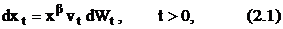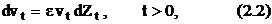and the initial conditions: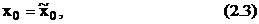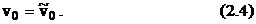The variable xt represents the forward prices/rates at time t, t>0, and vt is the stochastic volatility variable associated to xt at time t, t>0. The parameters b  and e are called respectively b-volatility of the forward prices/rates variable and volatility of volatility. We assume 0<b<1 and e>0. The stochastic processes Wt, Zt, t>0, are standard Wiener processes, such that W0=Z0=0 and dWt, dZt, t>0, are their stochastic differentials. The stochastic differentials dWt, dZt, t >0, are assumed to satisfy the condition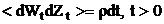, where <.> denotes the expected value of . and rÎ(-1,1). That is the stochastic differentials of dWt, dZt, t>0,  are assumed to be correlated with correlation coefficient rÎ(-1,1). The initial conditions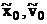are random variables that are assumed to be concentrated in a point with probability one and, for simplicity, we identify them with the points where they are concentrated. Moreover we assume>0. In order to guarantee that for t>0 the price variable xt is nonnegative and that the no arbitrage condition holds we impose an absorbing barrier in zero to the forward prices/rates variable xt, t>0.  That is we impose that the paths of the stochastic process xt, t>0, after going through xt=0 are no longer considered.

For m = 1,2,¼ let  Mm be the m-th moment with respect to zero of xt, t >0, we have: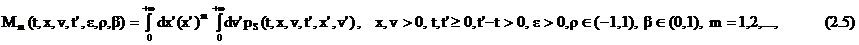where pS is the transition probability density function associated to the stochastic processes xt, vt, t >0, implicitly defined by (2.1), (2.2), (2.3), (2.4) and by the absorbing barrier in zero imposed to xt, t>0. Let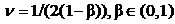, in  it has been shown that: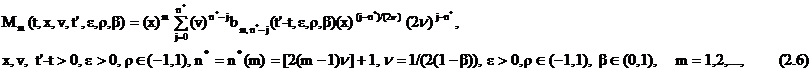where [.] denotes the integer part of . and the functions bj,m, j=0,1,2,…,n*, m=1,2,…, are defined by the following recursive relation: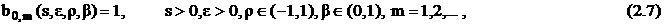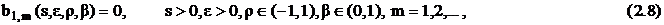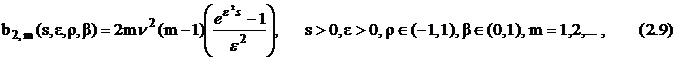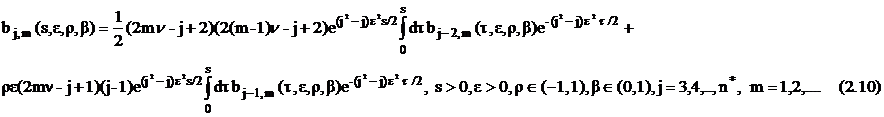The integrals that appear in (2.10) are elementary integrals that can be computed explicitly using the following formula:Formula (2.6) that gives the moments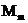,  m=1,2,..., of the forward prices/rates variable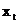, t>0,  is an explicit formula expressed using only elementary functions that does not contain integrals or series expansions. Formula (2.6)  is a new formula that can be used in many circumstances. For example it can be used in the formulation of calibration problems for the SABR model. In fact formula (2.6), thank to its closed form character and to the fact that contains only elementary functions can be evaluated very efficiently. In ,  formulae analogous to (2.6) have been used in the study of calibration problems for the normal and the lognormal SABR models.

## 3. The moments of the forward prices/rates variable: an interactive application and an app

We provide an interactive application and an app that given the values of the parameters of the SABR model, a time value t >0 and a moment order m (0<m£5) give the m-th order moment Mm at time t computed using formula (2.6) and using the Monte Carlo method. These two values of the same quantity  can be compared. In the interactive application and in the app the user can choose the size of the sample used in the Monte Carlo computation of the moment.  A set of default values of the parameters of the SABR model needed in the interactive application and in the app is given. The app is a simple translation of the interactive application in the Android environment. The app is freely downloadable  and runs on devices based on the Android software system.  For example it can be used on  Android smart phones and tablets.

INTERACTIVE APPLICATION

## 4 An explicit formula of the transition probability density function of the model when r=0 and the problem of probability conservation (movie 1)

Let us give an expression of the transition probability density function of the variables xt, vt, t>0, implicitly defined by (2.1), (2.2), (2.3), (2.4) when the correlation coefficient r is zero. Let us define the stochastic process:

 xt = xt1-b 1-b ,   t > 0,  b Î [0,1).

(4.1)

From Ito's lemma and equations (2.1), (2.2) it follows that the stochastic processes xt, vt, t > 0, satisfy the following system of stochastic differential equations:

 dxt = - b 2(1-b) 1 xt vt2dt+vt dWt, t > 0,

(4.2)

 dvt = e vt dZt,        t > 0.

(4.3)

The initial conditions (2.3), (2.4) become: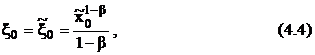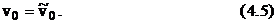An absorbing barrier in zero is imposed to the stochastic process xt, t > 0. The barrier imposed to xt, t > 0, comes from the barrier imposed to xt, t > 0.

Let pS(t,x,v,t¢,x¢,v¢), x, x¢, v, v¢, t, t¢ > 0, t¢-t > 0, be the transition probability density function of model (2.1), (2.2), (2.3), (2.4) with the absorbing barrier in zero imposed to xt , t>0, that is let pS(t,x,v,t¢,x¢,v¢), x, x¢, v, v¢, t, t¢ > 0, t¢-t > 0, be the probability density function of having xt¢ = x¢, vt¢ = v¢ given the fact that we have xt = x, vt = v when t¢-t > 0. Moreover let p(t,x,v,t¢,,v¢), x, , v, v¢, t, t¢ > 0, t¢-t > 0, be the transition probability density function of model (4.2), (4.3), (4.4), (4.5) with the absorbing barrier in zero imposed to xt , t>0, that is let p(t,x,v,t¢,,v¢), x, , v, v¢, t, t¢ > 0, t¢-t > 0, be the probability density function of having xt¢ = , vt¢ = v¢ given the fact that we have xt = x, vt = v when t¢-t > 0. We have:

 pS(t,x,v,t¢,x¢,v¢)dx¢ dv¢  =  p(t,x,v,t¢,x¢,v¢)dx¢ dv¢

 x = x1-b 1-b , x¢ = x¢1-b 1-b ,  x, x¢, v, v¢, t, t¢ > 0, t¢-t > 0.

(4.6)

The function p is the solution of the backward Kolmogorov equation associated to (4.2), (4.3), that is: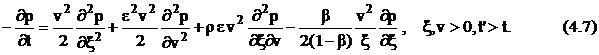with final condition:

 p(t¢,x,v,t¢,x¢,v¢) = d(x-x¢) d(v-v¢),  x,x¢,v,v¢ > 0,

(4.8)

and boundary condition:

 p(t,0,v,t¢,x¢,v¢) = 0,  x¢,v,v¢ > 0,  t¢ > t > 0,

(4.9)

where d is the Dirac's delta. The Dirichlet boundary condition (4.9) imposed to the function p is the condition  that  translates to p the condition imposed prescribing the absorbing barrier in zero  to the stochastic process xt, t>0. Note that p does not depend from t and t¢ separately, it depends only from s = t¢-t > 0. Let us introduce the function p*(s,x,v,,v¢) = p(t,x,v,t¢,,v¢), where s = t¢-t, x, v, v¢, t, t¢ > 0. From (4.7), (4.8), (4.9) it follows that p* is the solution of the partial differential equation: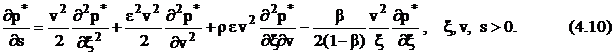with initial condition: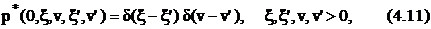and boundary condition: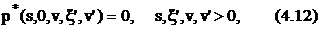Let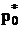be the transition probability density function p* when r=0. From (4.10), (4.11), (4.12) it follows that the function p*0 satisfies the partial differential equation: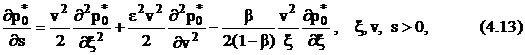with initial condition: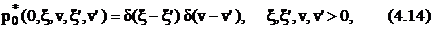and boundary condition: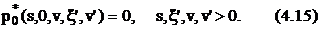In  it is shown that the solution of problem (4.13), (4.14), (4.15) is given by: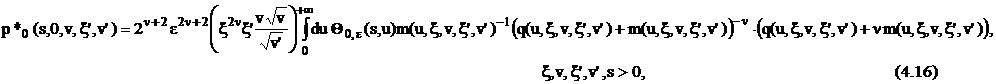where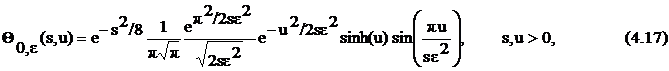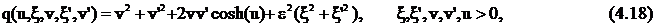and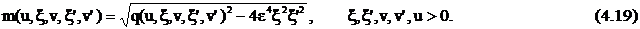It is easy to see that the function q(u,x,v, x’,v’)2-4e4x2x2 is positive for u>0,  x, x’, v, v’ > 0.  Note that the function p*0 defined in (4.16) satisfies the boundary condition (4.9). In fact when x = 0 the term x2n is zero and the functions q and m are “well behaved” in v, v’ for v, v’ > 0, x’, u >0.

Let s=t’-t>0,  t=0 and let “year” be the time unit on the t axis. In  MOVIE 1 we show the integral on [0,+¥)´[0,+¥) of the probability density function p*0 with respect to the future variables x’, v’ as a function of the past variables x, v,  for two values of  b=0.1, 0.5 when e=0.6, r=0 and the time varies from s=0 years to s=10 years. That is for the choices of the parameters specified previously we show the quantity: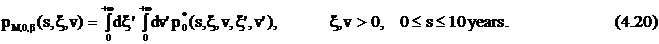In the models where the probability is conserved the function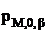defined in (4.19)  is  equal to one for every x, v, s > 0. This is not the case in the SABR model studied here. Note that we have chosen t=0 so that the past variables x, v  corresponds to  x0, v0 assigned in  (4.4), (4.5). MOVIE 1 shows that when time goes from s=0 to s=10 years there is an increasing loss of probability. Furthermore it is shown that  the loss of probability observed for b=0.5 is larger than the loss of probability observed for b=0.1.

## 5 Calibration and option prices forecast (movie 2)

Let us present a numerical experiment. In the experiment we consider the daily values of the futures price of the EUR/USD currency's exchange rate having maturity September 16th, 2011, (the third Friday of September 2011) (ticker YTU1 Curncy of Figure 1) and the daily prices of the corresponding European call and put options with expiry date September 9th, 2011 and strike prices  Ei = 1.375+0.005*(i-1), i = 1,2,¼,18. The strike prices Ei, i = 1,2,¼, 18, are expressed in USD. These prices have been observed in the time period that goes from September 27th, 2010, to July 19th, 2011. The observations are made daily and the prices considered are the closing prices of the day at the New York Stock Exchange. Recall that a year is made of about 250-255 trading days and that a month is made of about 20-22 trading days. Figure1 shows the futures price (ticker YTU1 Curncy) (blue line) and the EUR/USD currency's exchange rate (pink line) as a function of time. Figures 2 and 3 show respectively the prices (in USD) of the corresponding call and put options with maturity time September 9th, 2011 and strike price Ei, i = 1,2,¼,18, as a function of time.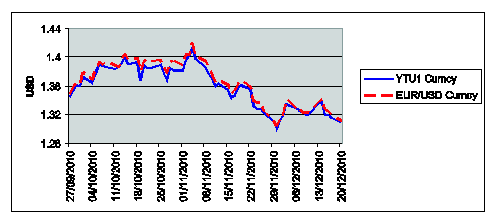Figure 1: YTU1 (blue line) and EUR/USD currency's exchange rate (pink line) versus time.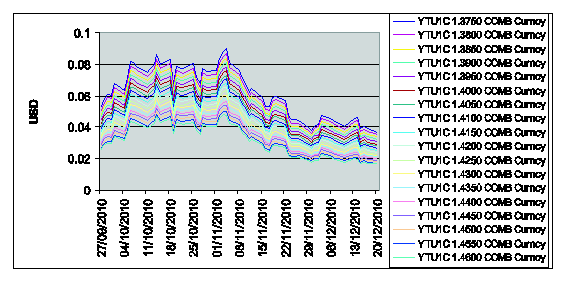Figure 2: Call option prices on YTU1 with strike price Ei = 1.375+0.005*(i-1), i = 1,2,¼,18, and expiry date T= September 9th, 2011 versus time.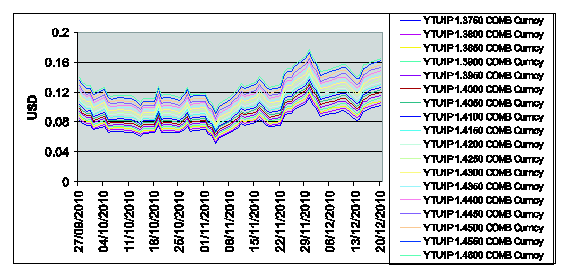Figure 3: Put option prices on YTU1 with strike price Ei = 1.375+0.005*(i-1), i = 1,2,¼,18, and expiry date T= September 9th, 2011 versus time.

We use the SABR model to study the data shown in Figures 1, 2, 3. Let R5 be the five-dimensional real Euclidean space, we introduce the vector Q = (r, e, b,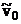, r) Î R5 and the set  MÌ R5 defined as follows: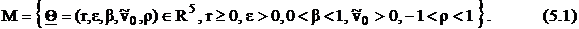The parameter r is the risk free interest rate that appears in the option pricing formulae derived in   and used in the calibration problem. We consider r as an unknown of the calibration problem. Note that sincecannot be observed in the financial markets it must be regarded as a parameter to be estimated in the calibration problem. The inequalities contained in (5.1) that define M are the natural constraints implied by the meaning in the model equations of the components of the vector Q. We use as data of the calibration problem the option prices observed at a given time (i.e. the option prices of Figures 2, 3 at a given date and we formulate the calibration problem as a nonlinear constrained least squares problem.

In the calibration problem studied the vector Q Î R5 is the unknown that is determined from the data (i.e. the futures EUR/USD prices and the option prices shown in Figures 1,2,3) and M is the set of the “feasible” choices of Q. This means that the solution of the calibration problem consists in finding the choice of Q ÎM that gives the best fit in the least squares sense between the observed option prices (i.e. the data) and the option prices obtained evaluating the formulae deduced in .

MOVIE 2  shows six animated windows. The upper left corner window shows the value of the futures prices of Figure 1 and the current time. The remaining five windows show the observed and forecast prices of the European call and put options for five values of the strike price (that is: E=E3 = 1.3850 (window (a)),   E=E5 = 1.3950 (window (b)), E= E7 = 1.4050 (window (c)), E= E9 = 1.415 (window (d)), E= E11 = 1.4250 (window (e))) are shown as a function of time. The forecasts shown in  MOVIE 2 are the forecasts one day-ahead of the day of observation of the option prices used to calibrate the SABR  model.

##  Fatone, L., Mariani, F., Recchioni, M.C., Zirilli, F.: The SABR model: explicit formulae of the moments of the forward prices/rates variable and  series expansions of the transition probability density and of the option prices, Journal of Applied Mathematics and Physics, 2, (2014), 540-568.

 Fatone, L., Mariani, F., Recchioni, M.C., Zirilli, F.: The use of statistical tests to calibrate the normal SABR model, Journal of Inverse

and Ill Posed Problems, 21(1),  (2013), 59-84,

 Fatone, L., Mariani, F., Recchioni, M.C., Zirilli, F.: Closed form moment formulae for  the lognormal SABR model and applications

to calibration problems, Open Journal of Applied Sciences, 3, (2013) , 345-359

Entry n. 1403